﻿ Calculation of Flows Intensities Transformations in Acyclic Directed Networks

Like us on:

# Calculation of Flows Intensities Transformations in Acyclic Directed Networks

Guram Tsitsiashvili*
Institute of Applied Mathematics Feb RAS, Far Eastern Federal University, Russia
*Corresponding Author: Guram Tsitsiashvili, Institute of Applied Mathematics Feb RAS, Far Eastern Federal University, Russia, Tel: +7 423 243-34-72, Email: [email protected]

Received Date: Nov 02, 2017 / Accepted Date: Nov 15, 2017 / Published Date: Nov 27, 2017

In this paper, a problem of calculation of signals or matters flows intensities in acyclic network is considered and sequential algorithm of its solution is suggesting.

The problem considered in this paper is discussing last year’s sufficiently intensively in different biological journals [1-3]. In Tsitsiashvili  a problem of a decomposition of balance equations for flows intensities in queuing networks is solving. Such problem is connecting with calculation of flows intensities of proteins networks. Main idea of this procedure is to find clusters with cyclically equivalent nodes and order them by their maximal distance from input clusters so that direct edges may be only from clusters with smaller to clusters with larger distances. Therefore, it is possible to divide all nodes into sets A0,A1,…,Ap so that every edge of the graph is only from node iAt to node jAq, t<q. Then a solution of balance equations system is dividing into solutions of sub systems for clusters with cyclically equivalent nodes so that these sub systems may be solving sequentially in accordance with their ordering by maximal distances.

However, in queuing theory this problem is connecting with flows of identical customers while in protein networks there are complex transformations of different matters and signals flows. Then at any step of calculations, it is possible to describe in every node j=0,1,…,m, of the network transformation of input signals and/or matters flows intensities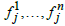into output signals and/ or matters flows intensities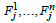. Here the node j=0 is as source so drain. This transformation is defining by some vector function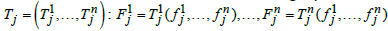. In simplest cases, this function may be defined using stoichiometric coefficients of some chemical reaction and the principle of limiting factors .

Suggesting procedure creates acyclic directed graph nodes, which are clusters of initial directed graph with cyclically equivalent nodes. If we deal with customers (signals) in queuing networks and know input flow intensity then it is possible to calculate output flows intensities for all nodes by solution of a system of linear algebraic equations. These systems of equations are to be solving in accordance with ordering of clusters. However, if we deal with acyclic network then these calculations are very simple to find the distribution of the input stream between network nodes in accordance with routing matrix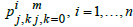.

Assume that in our network with finite number of nodes, 0,1,…,m, flows 1,…,n migrate and each kind i of flows moves along acyclic network in accordance with the route matrix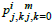so that if node jAq then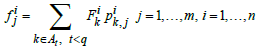. Denote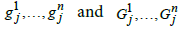proportions of signals or matters flows describing interaction (reaction) between signals or matters at node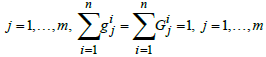. Then the function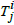, defining this interaction (reaction), has the form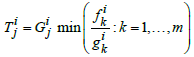where we put formally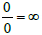. It is necessary to remark that parts of flows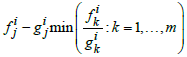are removed from the network (are directed to the node 0). At the node, 0 we know input flows intensities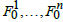. In conclusion, it is worthy to say that in this paper ordering of different network nodes is connecting with calculation of flows transformation in single node based on limiting factor. Last procedure may be considering in form that is more complicated.

To realize this procedure it is necessary to use sufficiently developed database of protein networks and sufficiently powerful computer.

The author thanks Bulgakov VP for useful recommendations.

#### References

Citation: Tsitsiashvili G (2017) Calculation of Flows Intensities Transformations in Acyclic Directed Networks. J Biom Biostat 8: 379. DOI: 10.4172/2155-6180.1000379

Copyright: © 2017 Tsitsiashvili G. This is an open-access article distributed under the terms of the Creative Commons Attribution License, which permits unrestricted use, distribution, and reproduction in any medium, provided the original author and source are credited.

Select your language of interest to view the total content in your interested language

##### Recommended Journals
Viewmore
###### Article Usage
• Total views: 1193
• [From(publication date): 0-2017 - Dec 07, 2019]
• Breakdown by view type
• HTML page views: 1140Can't read the image? click here to refresh